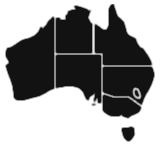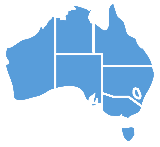Keywords: number, figure, numbered

## Sign Definition

### As a Noun

1. Any of the signs such as 'two', 'nine', 'eleven' or the written symbols such as 1, 3, 47. You use them to say how many things you are referring to or where something comes in a series. English = number, figure.
2. Any of the ten written symbols from 0 to 9 that are used to represent a number. English = figure.

### As a Verb or Adjective

1. To give something a number in a series and write that number on it, for example putting the numbers on the pages of a letter or putting numbers on tickets. English = number.
2. Of something, to be given a number in a series. English = (be) numbered.